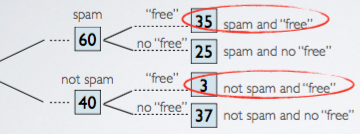### Introduction

Calculating probabilities can be hard, often it is hard to figure out what to do . But tree diagrams might be useful to the rescue!

In this section, we will introduce probability trees to solve for conditional probabilities. Highlight that they are especially useful when the probability we are asked for is the reverse of what we are given.

`P(A | B) → P(B | A)`

The probability tree is introduced through examples.

### Probability Tree from Examples

#### Example 1

Study: Spam probability

100 emails in inbox: 60 are spam, 40 are not.

Of the 60 spam emails, 35 contain the word "free". Of the rest, 3 contain the word "free".

If an email contains the word "free", what is the probability that it is spam?

The information is organized into a probability tree shown as below:The question is, if an email contains the word "free", what is the probability that it is spam? The email contain word "free" is going to be the given, and we are asked for the probability that is spam. So this is denoted as:

```                      35
P(spam | "free") = ---------- = 0.92
35 + 3```

#### Example 2

As of 2009, Swaziland had the highest HIV prevalence in the world. 25.9% of this country's population is infected with HIV. The ELISA test is one of the first and most accurate tests for HIV. For those who carry HIV, the ELISA test is 99.7% accurate. For those who do not carry HIV, the test is 92.6% accurate.

If an individual from Swaziland has tested positive, what is the probability that he carries HIV?

From the study, we know :

• The probability of having HIV is 0.259.
`P(HIV) = 0.259`
• The probability of testing positive given HIV is 0.997.
`P(+ | HIV) = 0.997`
• The probability of testing negative given no HIV is 0.926.
`P(- | no HIV) = 0.926`

In addition, we found the accuracy of the test is dependent on whether the patient has the disease. This conclusion can be explained by:

`P(+ | HIV) ≠ P(+ | no HIV)`

The question says, if an individual from Swaziland has tested positive, what is the probability that he carries HIV? This question can be denoted as:

`P(HIV | +) = ?`
```
P(+ | HIV) = 0.997 → P(HIV and +) = P(HIV) × P(+ | HIV)
/                                  = 0.259 × 0.997 = 0.2582
P(HIV) = 0.259
/               \
/                 P(- | HIV) = 0.003 → P(HIV and -) = 0.259 × 0.003
/                                                    = 0.0008

\
\                 P(+ | no HIV) = 0.074 → P(no HIV and +) = 0.741 × 0.074
\               /                                        = 0.0548
P(no HIV) = 0.741
\
P(- | no HIV) = 0.926 → P(no HIV and -) = 0.741 × 0.926
= 0.6862```

So, according to Bayes Theorem, the P(HIV | +) is:

```               P(HIV and +)          0.2582
P(HIV | +) = ---------------- = ----------------- = 0.82
P(+)           0.2582 + 0.0548```

Therefore, there is an 82% chance that individual from Swaziland who has tested positive actually carries HIV.